﻿ Mixed Constraints Cost Minimization Transportation Problem: An Effective Algorithmic ApproachAmerican Journal of Operational Research

p-ISSN: 2324-6537    e-ISSN: 2324-6545

2021;  11(1): 1-7

doi:10.5923/j.ajor.20211101.01

Received: Feb. 22, 2021; Accepted: Mar. 12, 2021; Published: Apr. 2, 2021### Mixed Constraints Cost Minimization Transportation Problem: An Effective Algorithmic Approach

Farhana Rashid1, Aminur Rahman Khan2, Md. Sharif Uddin2

1Department of Mathematics, Jagannath University, Dhaka, Bangladesh

2Department of Mathematics, Jahangirnagar University, Savar, Dhaka, Bangladesh

Correspondence to: Farhana Rashid, Department of Mathematics, Jagannath University, Dhaka, Bangladesh.
 Email: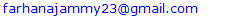Abstract

In the literature, there are several methods for finding an initial basic feasible solution to the cost-minimizing transportation problem with equality constraints. In this paper, we proposed an efficient algorithm for solving transportation problems in which the origin and destination constraints consist not only of equality but also inequality. The proposed method is easy to understand and to apply for finding an initial basic feasible solution to transportation problems happening in real-life situations.

Keywords: Transportation problem (TP), Cost Minimization Transportation Problem with Mixed Constraints (CMTP-MC), Initial Basic Feasible Solution (IBFS)

Cite this paper: Farhana Rashid, Aminur Rahman Khan, Md. Sharif Uddin, Mixed Constraints Cost Minimization Transportation Problem: An Effective Algorithmic Approach, American Journal of Operational Research, Vol. 11 No. 1, 2021, pp. 1-7. doi: 10.5923/j.ajor.20211101.01.

### 1. Introduction

The transportation problem (TP) is a special class of linear programming problems, which deals with shipping commodities from sources to destinations. The transportation problem is to find the amount of commodity which should be transported from each source to each destination satisfying all the supply and demand limits of sources and destinations respectively so that the overall transporting cost is minimum which the objective of the TP. If the total supply and demand limits are equal, then the problem is said to be a balanced transportation problem with equality constraints.
The transportation problem was formalized by the French Mathematician Monge, . Major advances were made in the field during World War II by the Russian Mathematician and Economist Leonid Vitaliyevich Kantorovich . The standard form of the transportation problem was first presented by Frank Lauren Hitchcock . Efficient methods of solution were derived by T. C. Koopman , Dantzig, G.B., , and then by Charnes A. et al. [6-8].
Many researchers developed a lot of methods to solve the Transportation problem. Some recent research work I mentioned here. Babu et al.  demonstrate that Vogel’s Approximation Method (VAM) has some limitations and computational blunders. To overcome these limitations, they developed an Improved Vogel’s Approximation Method (IVAM) by correcting these blunders.
Baidya  develop the stochastic solid transportation models to minimize the total transport time and ensuring safety with the safety and time objective functions. Dash and Mohanty et al.  inspect the TP where unit transportation cost, supplies, and demands are measured as uncertain normal variables. They develop the conceptual uncertain programming model for TP which is converted to a deterministic linear programming model. Sometimes per unit transport cost is uncertain due to the unstable condition of the fuel market. Gupta and Arora  provide an algorithm for determining the minimum unit transportation cost in a capacitated transportation problem with bounds on rim conditions. Ahmad and Adhami  develop a couple of mathematical optimization models to obtain the lower and upper bounds of the TP, where the supplies and demands quantities vary in an interval. Some transport systems impose a certain charge to use their routes along with the transport cost. Khurana and Adlakha  propose an algorithm for solving a multi-index fixed charge bi-criterion TP which minimizes the variable and fixed costs simultaneously to yield an optimal basic feasible solution. They also offer an algorithm to obtain an initial basic feasible solution of the multi-index TP.
A literature search uncovered that much effort has been focused on classical transportation problem with equality constraints which is computationally easy to solve. Very few numbers of papers observed in the literature address the transportation problem with mixed constraints. In our proposed method we solve the transportation problem with mixed constraints by adding one additional row and a column. Then step by step complete the total procedure. Our method is easy to understand gives a better result than another existing method. Then we provide a table showing the comparison of some existing methods and the proposed method. we proposed only the solution procedure of transportation problem with mixed constraints without touching MFL paradoxical situation.

### 2. Formulation of a General Transportation Problem

A set of m supply points (origins) O1, O2, … …, Oi, … …, Om from which a good is shipped. The supply point i can supply at most ai units. A set of n demand points (destinations) D1, D2, … …, Dj, … …, Dn to which the good is shipped. Demand point j must receive at least bj units of shipped goods. cij is the unit transportation cost and xij is the amount of product transported from sources i destinations j.
Using the above notation, the general transportation problem can be represented by the network diagram shown in Fig-1: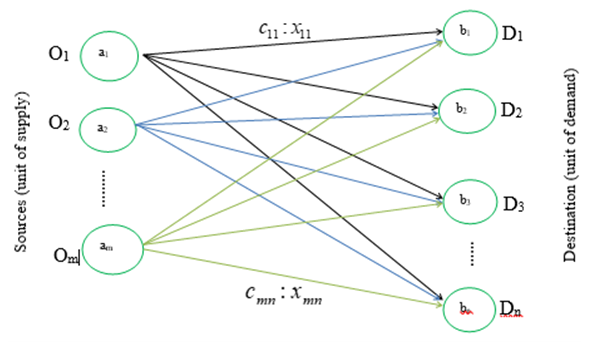Figure 1. Network Representation of Transportation Problem
We can also represent the TP by using matrix form as follows:
 Table 1. Matrix Representation of Transportation Problem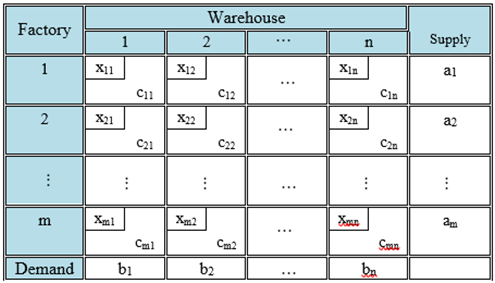Mathematically (For General case):
xij = number of units shipped from supply point i to demand point j.
Then the general formulation of a transportation problem is:
Minimize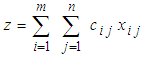subject to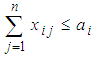;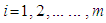(Supply constraints)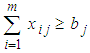;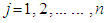(Demand constraints)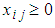. for all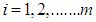and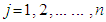.
In the case of Balanced TP may be written as
Minimize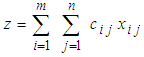.
subject to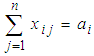;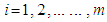(Supply constraints)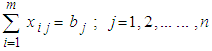(Demand constraints)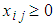; for all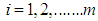and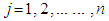.

### 3. Formulation of Transportation Problem with Mixed Constraints

Transportation problem with mixed constraints can be described as follows:
Suppose there are m origins Oi (iS={1,2,3,………m}) and we partitioned them into three sets S1, S2, S3. The set S1 must distribute exactly ai units of supply, S2 must distribute at least ai units of supply and S3 must distribute at most ai units of supply. Also, there are n destinations Dj (jT={1,2,3,………n}) and we partitioned them into three sets T1, T2, T3. The set T1 must receive exactly bi units, the set T2 must receive at least bi units and the set T3 must receive at most bi units of demand. The objective is to determine the amount xij transported from source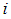to destination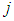while minimizing the total transport cost when satisfying mixed type supply and demand constraints.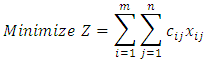Subject to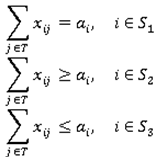and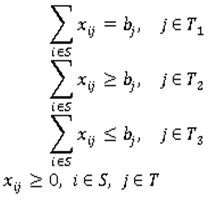We can also represent the above Transportation Problem with Mixed Constraints (TP-MC) by matrix form as follows:
 Table 2. Matrix Representation of Transportation Problem with mixed constraints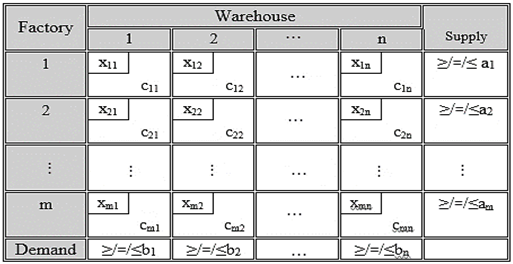### 4. Proposed Algorithm

The proposed algorithm for determining the initial solution consists of the following steps:
Step 1: Construct the transportation table with mixed constraints for the given problem.
Step 2:
We convert the TP with mixed constraints into Equivalence Standard Balance Transportation Problem (ESBTP) by introducing dummy row and column with cost 1 against the equal (=) and at least (≥) type constraints and cost 0 against the at most (≤) type constraints.
Also setting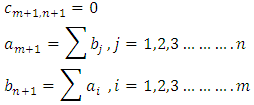Finally, we get balance TP with equality constraints.
Step 3:
Solve the ESBTP can be solved by using Vogel’s approximation method (VAM).
Step 4:
Shift the allocation from dummy cell to original cell by using the transformation: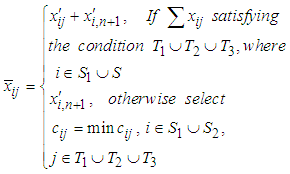And Continue this process until all allocations are satisfied for all row and similarly for a column that is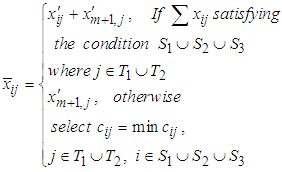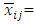optimal solution of the original problem,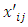= optimal solution of ESBTP.
Step 5:
Finally, compute the total transportation cost for the feasible allocations as the sum of the product of cost and corresponding allocated value of the transportation matrix and total unit of flow.
Remarks:
We consider Problem TP is bounded. Necessary and sufficient conditions for the existence of a feasible solution are found in Brigden (1974). Problem TP will be unbounded if there exists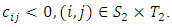### 5. Numerical Illustration

Example 1
There are three factories and four warehouses and the factory produces a homogeneous product. Factory A has a production capacity of exactly 20 units, Factory B has a production capacity of at least 16 units and Factory C has a production capacity at most 25 units. Likewise, Warehouse 1 having a capacity of demands at least 11 units, Warehouse 2 having a capacity of demands at most 13 units, warehouse 3 having a capacity of demands at least 17 units. Warehouse 4 having a capacity of demands exact 14 units. If per unit shipping cost from each factory to each warehouse is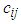which are given. Then this type of TP is called Transportation problem with mixed constraints and we conclude the minimum transportation cost with shipping units (flow) using our proposed method:
Step-1: Formulation –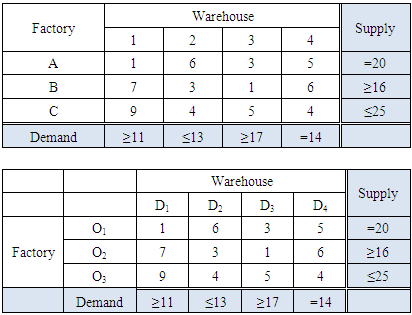Step-2: Convert TP-MC to Equivalence Standard Balance Transportation (ESBTP) Problem: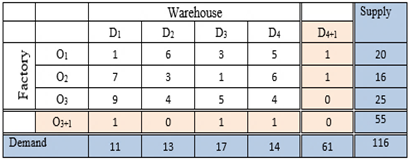Step-3 This is a balanced transportation problem. We solve it by using Vogel’s approximation method (VAM) and we get the allocation below: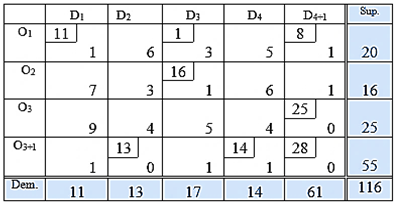Step-4: Now using transformation we get our required solution. Which is: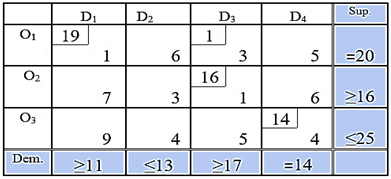Step-5: So required allocations are: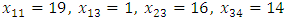, Total unit of flow-50
Total Cost =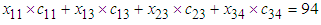.
The rest of the three examples are given below for making a comparison.
Example 2: [Ref: Klingman, D. et al., (1974)]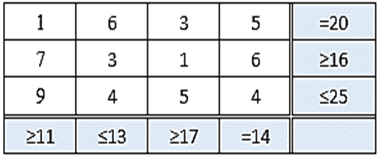Example 3: Ref: Mondal, R.N. et al., (2015)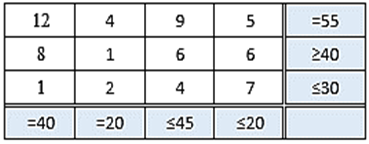Example 4: Ref: Panadian, P. et al., (2010)]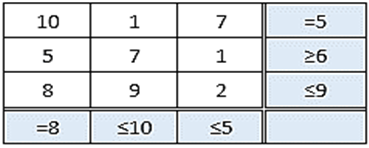### 6. Comparative Study

The accompanying Table-3 demonstrates an examination for expense alongside the total unit of flow. Arrangements got by our proposed calculation and the other well-known existing calculations and Table-4 speak to the per-unit cost from where we effortlessly distinguish the best calculation.
 Table 3. A Comparative Study of Different Solutions Shows cost and flow of the unit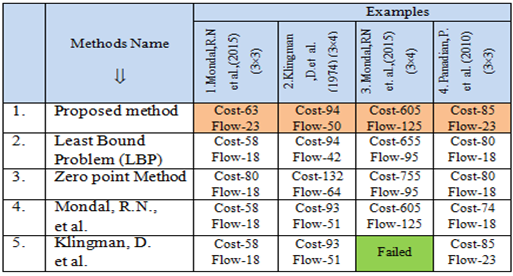Table 4. Comparative study shows per unit cost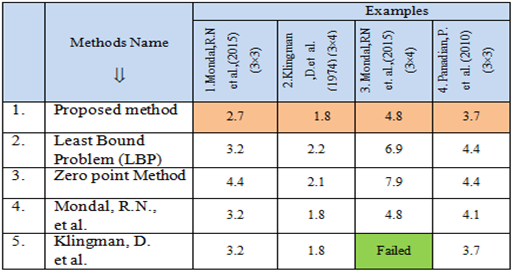### 7. Conclusions

In this paper, we have developed an effective algorithm for solving transportation problems with mixed constraints. A comparative study is carried out here on twenty randomly selected problems of a different order from some reputed journals. This comparison shows that our proposed method gives a better outcome over other existing calculations and we trust this new idea will help individuals who are working in this field. Still, there exists a rich opportunity for further research in this subject.